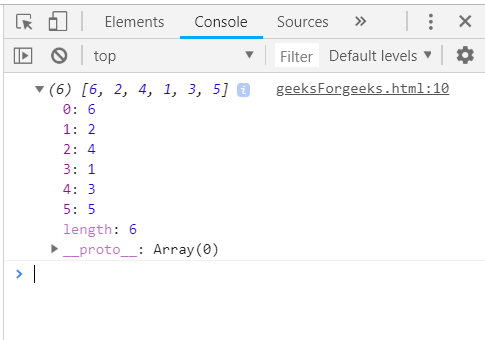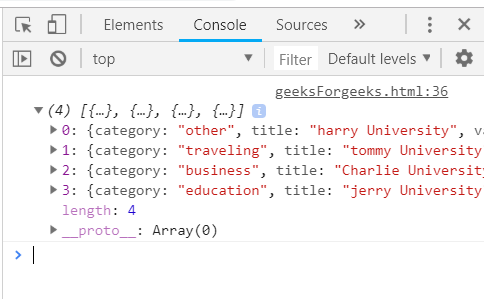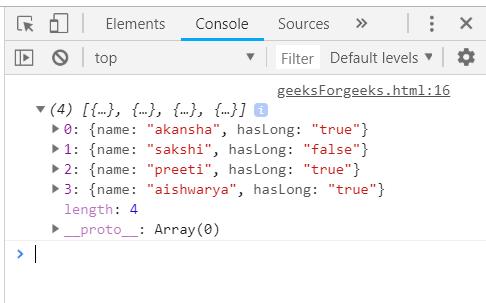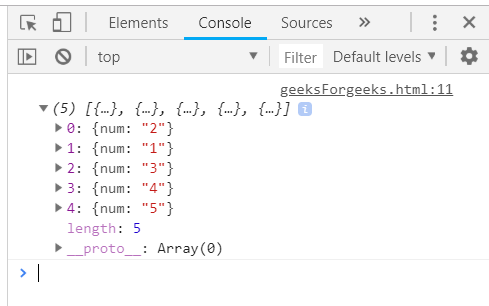Related Articles
Underscore.js | shuffle() with Examples
• Last Updated : 17 Oct, 2019

The Underscore.js is a JavaScript library that provides a lot of useful functions that helps in the programming in a big way like the map, filter, invoke etc even without using any built-in objects.
The _.shuffle() function is used to arrange a list of array in random manner. This _.shuffle() underscore function uses Fisher Yates Shuffle which is discussed in the below mentioned article. So, every time we use this the output of this function will be different according to the Fisher Yates Shuffle.

Syntax:

`_.shuffle(list)`

Parameters: This function accepts single parameter List. This parameter is used to hold the list of items which will be shuffled.

Return values: The returned value is the new randomized array containing all the elements which are in the original array which as passed to the _.shuffle() function.

Passing a numeric array to the _.shuffle() function: The ._shuffle() function takes the element from the list one by one and do the specified operations according to the fisher Yates Shuffle. Then console.log() the final answer which will contain all the elements of the original array in the randomized problem.

Example:

 `<``html``> ` `    ``<``head``> ` `        ``<``script` `src` `=  ` `        ``"https://cdnjs.cloudflare.com/ajax/libs/underscore.js/1.9.1/underscore-min.js"` `> ` `        `` ` `    `` ` `    ``<``body``> ` `        ``<``script` `type``=``"text/javascript"``> ` `            ``console.log(_.shuffle(_.shuffle([1, 2, 3, 4, 5, 6]))); ` `        `` ` `    `` ` `                     `

Output:Passing a structure to _.shuffle() function: Passing a structure to the _.shuffle() function. First declare the array like here the array is ‘goal’ and then pass this array to the _.shuffle() function. The elements of the ‘goal’ array will be shuffled along with all their properties.

Example:

 `<``html``> ` `    ``<``head``> ` `        ``<``script` `src` `=  ` `        ``"https://cdnjs.cloudflare.com/ajax/libs/underscore.js/1.9.1/underscore-min.js"` `> ` `        `` ` `    `` ` `    ``<``body``> ` `        ``<``script` `type``=``"text/javascript"``> ` `             ``var goal  = [ ` `            ``{ ` `                ``"category" : "other", ` `                ``"title" : "harry University", ` `                ``"value" : 50000, ` `                ``"id":"1" ` `            ``}, ` `            ``{ ` `                ``"category" : "traveling", ` `                ``"title" : "tommy University", ` `                ``"value" : 50000, ` `                ``"id":"2" ` `            ``}, ` `            ``{ ` `                ``"category" : "education", ` `                ``"title" : "jerry University", ` `                ``"value" : 50000, ` `                ``"id":"3" ` `            ``}, ` `            ``{     ` `                ``"category" : "business", ` `                ``"title" : "Charlie University", ` `                ``"value" : 50000, ` `                ``"id":"4" ` `            ``} ` `        ``] ` `        ``console.log(_.shuffle(goal)); ` `        `` ` `    `` ` ` `

Output:Passing a list with one property as true/false to the _.shuffle() function: First declare the array (here array is ‘people’). Choose one condition on which need to check like here ‘hasLongHairs’. Console.log the final answer. The final answer will be a randomized array as the fisher yates shuffle uses a random function in it’s algorithm.

Example:

 `<``html``> ` `    ``<``head``> ` `        ``<``script` `src` `=  ` `        ``"https://cdnjs.cloudflare.com/ajax/libs/underscore.js/1.9.1/underscore-min.js"` `> ` `        `` ` `    `` ` `    ``<``body``> ` `        ``<``script` `type``=``"text/javascript"``> ` `             ``var people = [ ` `            ``{"name": "sakshi", "hasLong": "false"}, ` `            ``{"name": "aishwarya", "hasLong": "true"}, ` `            ``{"name": "akansha", "hasLong": "true"}, ` `            ``{"name": "preeti", "hasLong": "true"} ` `        ``] ` `         ``console.log(_.shuffle(people, 'name')); ` `        `` ` `    `` ` ` `

Output:Declaring an array and then passing it to the _.shuffle() function: Declare an array let ‘users’ with a property as ‘num’ and then pass it to the _.shuffle() function. Then console.log the new randomized array. The output will be different every time you run it.

Example:

 `<``html``> ` `    ``<``head``> ` `        ``<``script` `src` `=  ` `        ``"https://cdnjs.cloudflare.com/ajax/libs/underscore.js/1.9.1/underscore-min.js"` `> ` `        `` ` `    `` ` `    ``<``body``> ` `        ``<``script` `type``=``"text/javascript"``> ` `             ``var users = [{"num":"1"}, {"num":"2"}, {"num":"3"}, {"num":"4"}, {"num":"5"}]; ` `        ``console.log(_.shuffle(users, 'id')); ` `        `` ` `    `` ` ` `

Output:My Personal Notes arrow_drop_up
Recommended Articles
Page :